Contents >> Applied Mathematics >> Matrix Algebra >> Principles of Matrix Calculation >> Cellular matrices

 Matrix Algebra - Cellular matrices Cellular matrices Let's consider some matrix A and we’ll split it into matrices of lower order: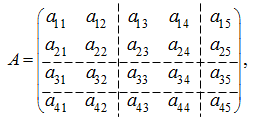which are called cells or blocks . Here cells (blocks) are matrices: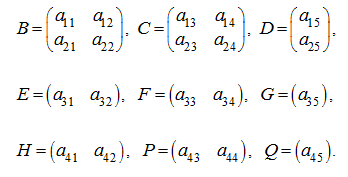Now the matrix A can be considered as cellular or block matrix: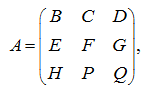which elements are cells (blocks). Apparently, that splitting of any matrix into cells (blocks) is maybe executed by various ways. In that specific case the cellular matrix can be quasi-diagonal one: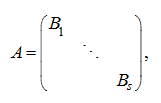where cells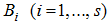– square matrices (generally speaking, of different orders), and outside of cells zeros are. Note, that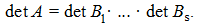Cellular matrices of the same dimensions and with identical splitting are called conform . Operations with cellular matrices are carried out by the same rules, as with usual matrices. 1. Addition and subtraction of cellular matrices Let there are two conform cellular matrices: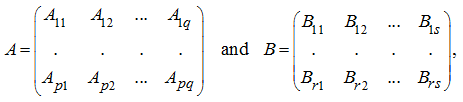where p = r , q = s and cells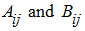of identical dimension. Then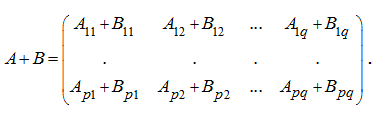Subtraction of cellular matrices is carried out similarly. 2. Multiplication of cellular matrices Multiplication of a cellular matrix to a number (scalar) Let A – a cellular matrix and h – a number, then we have: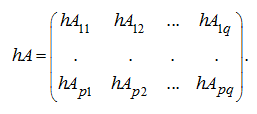Multiplication of cellular matrices Let's consider two conform cellular matrices:and q = r . Let all cells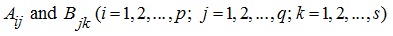such, that a number of columns of a cell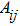is equal to a number of rows of a cell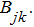(For example, apparently, that it takes place in that specific case, when all cells– square matrices and have also the same order). Then it is easy to show, that a product of matrices A and B is too a cellular matrix: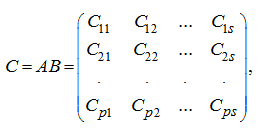where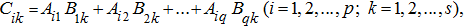that is multiplication of cellular matrices is similar to multiplication of numerical matrices . E x a m p l e .  Multiply the cellular matrices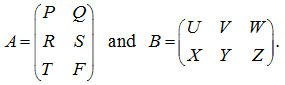S o l u t i o n .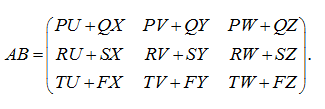< Previous Contents Next >